# Bihar Board 12th Physics Objective Answers Chapter 5 Magnetism and Matter

Bihar Board 12th Physics Objective Questions and Answers

## Bihar Board 12th Physics Objective Answers Chapter 5 Magnetism and Matter

Question 1.
The primary origin of magnetism lies in
(a) atomic current and intrinsic spin of electrons.
(b) polar and non polar nature of molecules.
(e) pauli exclusion principle.
(d) electronegative nature of materials.
(a) atomic current and intrinsic spin of electrons.Question 2.
Magnetic moment for a solenoid and corresponding bar magnet is
(a) equal for both
(b) more for solenoid
(c) more for bar magnet
(d) none of these
(a) equal for both

Question 3.
Which of the following is correct about magnetic
monopole?
(a) Magnetic monopole exist.
(b) Magnetic monopole does not exist.
(c) Magnetic monopole have constant value of monopole momentum.
(d) The monopole momentum increase due to increase at its distance from the field.
(b) Magnetic monopole does not exist.

Question 4.
The pole strength of 12 cm ¡ong bar magnet is 20 A m. The magentic induction at a point 10 cm away from
tue centre of the magnet on its axial line is $$\left[\frac{\mu_{0}}{4 \pi}=10^{-7} \cdot \mathrm{H} \mathrm{m}^{-1}\right]$$
(a) 1.17 ×  10-3T
(b) 2.20 × 10-3 T
(c) 1.17 × 10-2T
(d) 2.20 × 10-2T
(a) 1.17 × 10-3T
Solution:
(a) On axial line , $$B=\frac{\mu_{0}}{4 \pi} \frac{2 m d}{\left(d^{2}-l^{2}\right)^{2}}$$
Given, 21 = 12 cm = 0.12 m
Pole strength = 20 A m, d- 10 cm = 0.1 m
Magnetic moment m – 20 x 0.12 Am2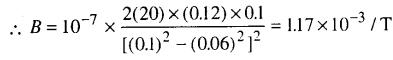Question 5.
What is the magnitude of axiai field due to a bar magnet of length 3 cm at a distance of 75 cm from its mid-point if its magnetic moment is 0.6 Am2?
(a) 0.013 µT
(b) 0.113 µT
(c) 0.213 µT
(d) 0.313 µT
(c) 0.213 µT
Solution:
(c) Here, m = 0.6 A m2, r- 75 cm = 0.75 m Mo∴ Baxial = 2.13 x 10-7 T = 0.213µT

Question 6.
A solenoid of cross-sectional area 2 × 10-4 m2 and 900 turns has 0.6 A m2 magnetic moment. Then the current flowing through it is
(a) 2.24 A
(b) 2.34 mA
(c) 3.33 A
(d) 3.33 mA
(c) 3.33 A
Solution:
(c) Here, N = 900 turns, A = 2 x 10-4 m2, ms = 0.6 A m2
The magnetic moment of solenoid ms = NIA
The current flowing through the solenoid is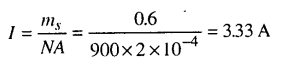Question 7.
A circular coil of 300 turns and diameter 14 cm carries a current of 15 A. The magnitude of magnetic moment
associated with the loop is
(a) 51.7JT-1
(b) 69.2 J T-1
(c) 38.6 J T-1
(d) 19.5 J T-1
(b) 69.2 J T-1

Question 8.
The torque and magnetic potential energy of a
magnetic dipole in most stable position in a uniform
magnetic field ($$\vec{B}$$) having magnetic moment ($$\vec{m}$$) will be
(a) -mB, zero
(b) mB. zero
(c) zero, mb
(d) zero, -mb
(d) zero, -mbQuestion 9.
The work done in moving a dipole from its most stable to most unstable position in a 0.09 T uniform magnetic field is (dipole moment of this dipole = 0.5 A m2)
(a) 0.07 J
(b) 0.08 J
(c) 0.09 J
(d) 0.01 J
(c) 0.09 J

Question 10.
The magnetic moment of a short bar magnet placed with its magnetic axis at 30° to an external field of 900 G and experience a torque of 0.02 N m is
(a) 0.35 A m2
(b) 0.44 A m2
(c) 2.45 Am2
(d) 1.5 Am2
(b) 0.44 A m2
Solution:
(b) Here, B = 900 Gauss = 900 x 10-4 T.
= 9 x 10-2 T
τ = 0.02 N m and θ = 30°
∴ τ = mB sin θ
⇒ 0.02 = m x 9 x 10-2 x sin 30°
0.02 = 9 x 10-2 x $$\frac{1}{2}$$ x m
m = $$\frac{0.02 \times 2}{9 \times 10^{-2}}$$ = 0.44Am2

Question 11.
The net magnetic flux through any closed surface, kept in a magnetic field is
(a) zero
(b) $$\frac{\mu_{0}}{4 \pi}$$
(c) 4πμ0
(d) $$\frac{4 \mu_{0}}{\pi}$$
(a) zero

Question 12.
The earth behaves as a magnet with magnetic field pointing approximately from the geographic
(a) North to South
(b) South to North
(c) East to West
(d) West to East
(b) South to NorthQuestion 13.
The strength of the earth’s magnetic field is
(a) constant everywhere
(b) zero everywhere
(c) having very high value
(d) vary from place to place on the earths surface
(d) vary from place to place on the earths surface

Question 14.
Which of the following is responsible for the earth’s magnetic field ?
(a) Convective currents in earth’s core.
(b) Diversive current in earth’s core.
(c) Rotational motion of earth.
(d) Translational motion of earth.
(a) Convective currents in earth’s core.

Question 15.
Which of the following independent quantities is not used to specify the earth’s magnetic field ?
(a) Magnetic declination (θ).
(b) Magnetic dip (δ).
(c) Horizontal component of earth’s field (BH).
(d) Vertical component of earth’s field (Bv).
(d) Vertical component of earth’s field (Bv).

Question 16.
The dip angle at a location in southern India is about 18°. Then the dip angle in Britain will be
(a) greater than 18°
(b) lesser than 18°
(c) equal to 18°
(d) zero
(a) greater than 18°

Question 17.
The angle of dip at a certain place where the horizontal and vertical components of the earth’s magnetic field are equal is
(a) 30°
(b) 75°
(c) 60°
(d) 45°
(d) 45°Question 18.
The vertical component of earth’s magnetic field at a place is √3 times the horizontal component the value of angle of dip at this place is
(a) 30°
(b) 45°
(c) 60°
(d) 90°
(c) 60°

Question 19.
The angles of dip at the poles and the equator respectively are
(a) 30°, 60°
(b) 0°, 90°
(c) 45°, 90°
(d) 90°, 0°
(d) 90°, 0°

Question 20.
The equatorial magnetic field of earth is 0.4 G. Then its dipole moment on equator is
(a) 1.05 x 1023 A m2
(b) 2.05 × 1023 A m2
(c) 1.05 × 1021 A m2
(d) 2.05 × 1021 A m2
(a) 1.05 × 1023 A m2
Solution: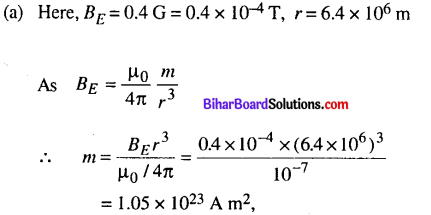Question 21.
A magnetising fieid of 1500 A m-1 produces flux of 2.4 × 10-5 weber in a iron bar of the cross-sectional area of 0.5 cm2. The permeability of the iron bar is
(a) 245
(b) 250
(c) 252
(d) 255
(d) 255
Solution:
(d) Here, H = 1500 A m-1 , Φ = 2.4 x 10-5
weber A = 0.5 cm2 = 0.5 x 10-4 m2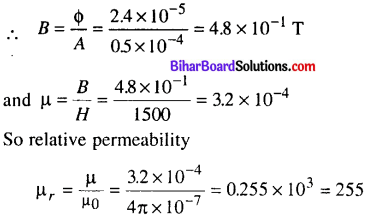Question 22.
A solenoid has a core of a substance with relative permeability 600. What is the magnetic permeability of the given substance ?
(a) 20π × 10-5 N A2
(b) 2lπ × 10-5 N A2
(c) 22π × 10-5 N A2
(d) 24π × 10-5 N A2
(d) 24π × 10-5 N A2
Solution:

(d) As μ = μr μ0
Here, μr = 600 and μ0 = 4π x 10-7 N A-2 Magnetic permeability, μ = 600 x 4π x 10-7 = 24π x 10-5 N A-2Solution:Question 23.
A permanent magnet in the shape of a thin cylinder of length 50 cm has intensity of magnetisation 106 A m-1. The magnetisation current is
(a) 5 × 105 A
(b) 6 × 105 A
(c) 5 × 104 A
(d) 6 × 104 A
(a) 5 × 105 A

Question 24.
A magnetising field of 2 × 103 A m-1 produces a magnetic flux density of 8 π T in an iron rod. The relative permeability of the rod will be
(a) 102
(b) 1
(c) 104
(d) 103
(c) 104
Solution:
(c) Here, H= 2 x 103 Am-1,
B = 8πT = μ0 = 4π x 10-7
Since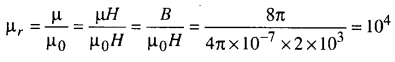Question 25.
The relation connecting magnetic susceptibility χm and relative permeability pr is
(a) χm = μr + 1
(b) μr = μr – 1
(c) $$\chi_{m}=\frac{1}{\mu_{r}}$$
(d) χm = 3(1 + μr)
(b) μr = μr – 1

Question 26.
The relative permeability of iron is 6000. Itsmagnetic susceptibility is
(a) 5999
(b) 6001
(c) 6000 × 10-7
(d) 6000 × 107
(a) 5999

Question 27.
Which of the following is universal magnetic property ?
(a) Ferromagnetism
(b) Diamagnetism
(c) Paramagnetism
(d) Anti-ferromagnetism
(b) DiamagnetismQuestion 28.
Magnetic susceptibility of a diamagnetic substances
(a) increase with increase in temperature
(b) increases with decrease in temperature
(c) remains constant with change in temperature
(d) none of these
(c) remains constant with change in temperature

Question 29.
A ball of superconducting material is dipped in liquid nitrogen and placed near a bar magnet. In which direction will it move ?
(a) Away from bar magnet
(b) Towards the bar magnet
(c) Around the bar magnet
(d) Remain constant
(a) Away from bar magnet

Question 30.
Out of given paramagnetic substance (Calcium, Chromium, Oxygen and Tungsten) which substance has maximum susceptibility ?
(a) Calcium
(b) Chromium
(c) Oxygen
(d) Tungsten
(b) ChromiumQuestion 31.
The correct M-H curve for a paramagnetic material at a constant temperature (T) is represented by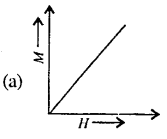Question 32.
The magnetic susceptibility of a paramagnetic material at -73“ C is 0.0075, its value at -173″C will be
(a) 0.0045
(b) 0.0030
(c) 0.015
(d) 0.00754
(c) 0.015
Solution:
(c) Here, χm = 0.0075,
T1 =-73 °C = (-73 + 273 ) K = 200 K
T2 = – 173 °C = (-173 + 273) K = 100 K, χm2 = ?
As for paramagnetic material magnetic susceptibility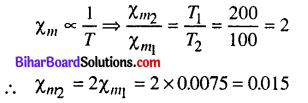Question 33.
The magnetic susceptibility of a paramagnetic sustance at -173° C is 1.5 × 10-2 then its value at -73 “C will be
(a) 7.5 × 10-1
(b) 7.5 × 10-2
(c) 7.5 × 10-3
(d) 7.5 × 10-4
(c) 7.5 × 10-3

Question 34.
A paramagnetic liquid is taken in a U-tube and arranged so that one of its limbs is kept between pole pieces of the magnet. The liquid level in the limb
(a) goes down
(b) rises up
(c) remains same
(d) first goes down and then rise
(b) rises upQuestion 35.
Mark the correct set of ferromagnetic substance
(a) iron, cobalt and nickel
(c) silicon, bismuth and nickel
(d) aluminium, sodium and copper.
(a) iron, cobalt and nickel

Question 36.
In an experiment it is found that the magnetic susceptibility of given sustance is much more greater than one. The possible substance is
(a) diamagnetic
(b) paramagnetic
(c) ferromagnetic
(d) nonmagnetic
(c) ferromagnetic

Question 37.
Magnetic permeability is maximum for
(a) ferromagnetic substances
(b) diamagnetic substances
(c) paramagnetic substances
(d) all of these
(a) ferromagnetic substances
Solution:
(c) In paramagnetic substance the magnetic susceptibility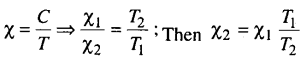Here, χ1= 15 x 10-2,
T1 = 273 – 173 = 100 K, T2 = 273 – 73 = 200 K
χ2 =1.5 x 10-2 x $$\frac{100}{200}$$ = 7.5 x 10-3Question 38.
Nickel shows ferromagnetic property at room temperature. If the temperature is increased beyond Curie temperature, then it will show
(a) anti ferromagnetism
(b) no magnetic property
(c) diamagnetism
(d) paramagnetism
(d) paramagnetism

Question 39.
The temperature of transition from ferromagnetic property to paramagnetic property is called
(a) Transition temperature
(b) Critical temperature
(c) Curie temperature
(d) Triplet temperature.
(c) Curie temperature

Question 40.
The hysteresis cycle for the material of a transformer core is
(a) short and wide
(b) tall and narrow
(c) tall and wide
(d) short and narrow
(b) tall and narrow

Question 41.
The magnetising field required to be applied in opposite direction to reduce residual magnetism to zero is called
(a) retentivity
(b) coercivity
(c) hysteresis
(d) flux
(b) coercivity

Question 42.
Which of the following material is used in making the core of a moving coil galvanometer ?
(a) Copper
(b) Nickel
(c) Iron
(d) Both (a) & (b)